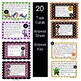# 4.OA.A.1,2,3 Halloween Multiplicative Comparison ReviewSubject
Resource Type
File Type

PDF

(2 MB|20 pages)
Product Rating
Standards
• Product Description
• StandardsNEW

This resource is contains 20 task cards that fourth graders can use to review multiplicative comparison, factors and multiples, the relationship between multiplication and division, and multi-step word problems.

Just print the cards, cut, and hang them around the classroom. Make copies of the student answer sheets for them to use to put their answers to each problem, and check with the included answer key. You may want to provide students with a treat or small prize for completing the task cards correctly.

I'm using the set for review before our chapter test, but I will also use them as review during the year from time to time.

Solve multistep word problems posed with whole numbers and having whole-number answers using the four operations, including problems in which remainders must be interpreted. Represent these problems using equations with a letter standing for the unknown quantity. Assess the reasonableness of answers using mental computation and estimation strategies including rounding.
Multiply or divide to solve word problems involving multiplicative comparison, e.g., by using drawings and equations with a symbol for the unknown number to represent the problem, distinguishing multiplicative comparison from additive comparison.
Interpret a multiplication equation as a comparison, e.g., interpret 35 = 5 × 7 as a statement that 35 is 5 times as many as 7 and 7 times as many as 5. Represent verbal statements of multiplicative comparisons as multiplication equations.
Total Pages
20 pages
Included
Teaching Duration
N/A
Report this Resource to TpT
Reported resources will be reviewed by our team. Report this resource to let us know if this resource violates TpT’s content guidelines.